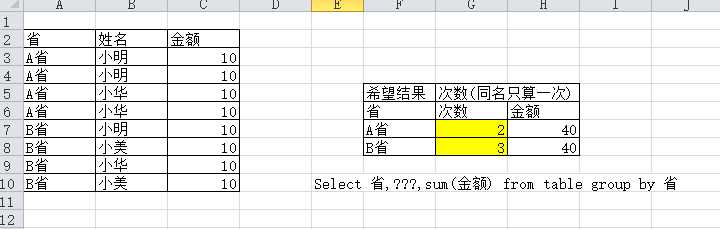mysql 简单的计数问题，一样姓名，不重覆计算 5C5个回答

``````select 省, min(姓名) as 姓名, count(姓名) as 次数, sum(金额) as 金额 from table group by 省
``````

``````select 省, count(姓名), sum(total) from (
select 省, 姓名, sum(金额) total from table group by 省,姓名
) a
group by 省

``````

select 省, count(distinct 姓名), sum(金额) from table group by 省,姓名